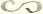## 3-15-40. George Howard Darwin to H. Poincaré

Oct. 22.01

Newnham Grange–Cambridge

Dear Monsieur Poincaré,

Your M.S. has arrived.11endnote: 1 Poincaré a annoncé l’envoi de son mémoire par lettre (§ 3-15-39). I shall venture to annotate it a little in red ink with instructions to the printers in English as I think it may save much trouble in the proofs. Of course I have experience of their ways which you cannot have.

I think it might be useful if I append a note in my own name to explain the identities of your $R$’s and my $\mathfrak{P}$ and $\mathbf{P}$. It has taken me nearly half an hour to make it out & I may as well save others the trouble. In doing this however I have discovered two things. I am unable to find any $R_{0}$ in the Acta, but I gather from your M.S. that it must denote $\mathfrak{P}_{0}(v)$ a constant or unity.

Secondly in yr explanation you do not really follow yr notation of the Acta.22endnote: 2 In the published memoir, Poincaré notes that he and Darwin do not employ the same notation, and that he employs a notation different from that employed in his Acta paper (1902, 335–336). You write there $R^{(k)}_{n,i}$ and $n$ is clearly the degree of the harmonic and $i$ its order, because you refer to its becoming

 $A(\rho^{2}-e^{2})^{\frac{1}{2}i}D^{i+n}(p^{2}-e^{2})^{n}$

in the case of the spheroid. Hence where in yr M.S. you write $R^{\prime}_{0,2}$ and $R^{\prime}_{0,3}$ you mean $R^{\prime}_{2,0}$ and $R^{\prime}_{3,0}$. Accordingly I propose (with your consent) to correct this.33endnote: 3 Darwin’s note was inserted in Poincaré’s paper (Poincaré 1902, 336). I then obtain the following for your new $R$’s.

 $\displaystyle R_{1}$ $\displaystyle=\mathfrak{P}_{0}(v),$ $\displaystyle R_{2}$ $\displaystyle=\mathfrak{P}^{1}_{1}(v),$ $\displaystyle R_{3}$ $\displaystyle=\mathfrak{P}_{2}(v),$ $\displaystyle R_{4}$ $\displaystyle=\mathfrak{P}^{2}_{2}(v),$ $\displaystyle R_{4}$ $\displaystyle=\mathfrak{P}_{3}(v),$

Of course if in any case you dislike the mode of printing which I shall suggest it will be open to you to correct it in proof.

I do not think our printers have any type for yourexcept one like this $\backsim$ which has been used by some writers to denote a difference without regard to sign. On the other hand we have $\propto$ which has been used as equivalent to “varies as” & this is your meaning. I suggest therefore $\propto$ to replace.

Our printers always make such a thing as $\frac{\xi}{2}$ look very ugly, & so I write $\frac{1}{2}\xi$. This moreover saves the compositor much trouble. I have suggested this in many places.

I am afraid the French will be very badly set up & so I will look over a first proof before sending it on to you. I will write again if anything occurs to me. I have not yet had time to master your method. I am besides very busy for some days to come.

Yours sincerely,

G. H. Darwin

ALS 4p. Collection particulière, Paris.

Time-stamp: " 4.05.2019 00:12"

### Notes

• 1 Poincaré a annoncé l’envoi de son mémoire par lettre (§ 3-15-39).
• 2 In the published memoir, Poincaré notes that he and Darwin do not employ the same notation, and that he employs a notation different from that employed in his Acta paper (1902, 335–336).
• 3 Darwin’s note was inserted in Poincaré’s paper (Poincaré 1902, 336).

## References

• H. Poincaré (1902) Sur la stabilité de l’équilibre des figures piriformes affectées par une masse fluide en rotation. Philosophical Transactions of the Royal Society A 198, pp. 333–373. Cited by: endnote 2, endnote 3.